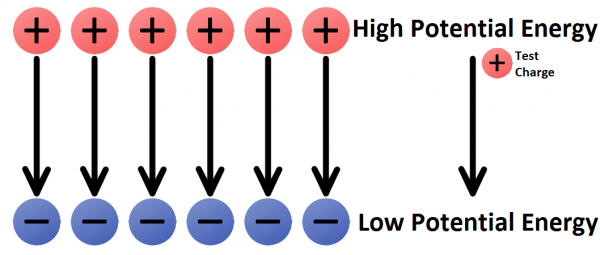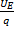# Electric Potential

Electric potential describes how much energy is stored in an electric field at a particular point. It is defined as the amount of electrical potential energy per unit charge at a particular point in the field.

It is important to remember that electric potential and electric potential energy are two different things. Electrical potential energy is the energy that a charged object has because of its position in an electric field. Electric potential is the amount of energy stored in an electric field at a particular point.

Electric potential is measured in joules per coulomb (i.e. energy per unit charge, J.C-1) and this is defined as a volt (V).

If we look at an electric field, we can consider two points:There will be a point of high potential - at this point, a positive charge has the highest potential energy - and there will be a point of low potential, where the charge would have the lowest potential energy. Thus, electric potential and electrical potential energy are linked.

If we consider the difference in electric potential between two points in an electric field, we would determine the voltage (V) of the system. Voltage gives an indication of the power or pressure of an electric field.

Incorporating all of this together we can represent electric potential with the following formula:

V =Where UE is electrical potential energy and q is a test charge in the electric field.